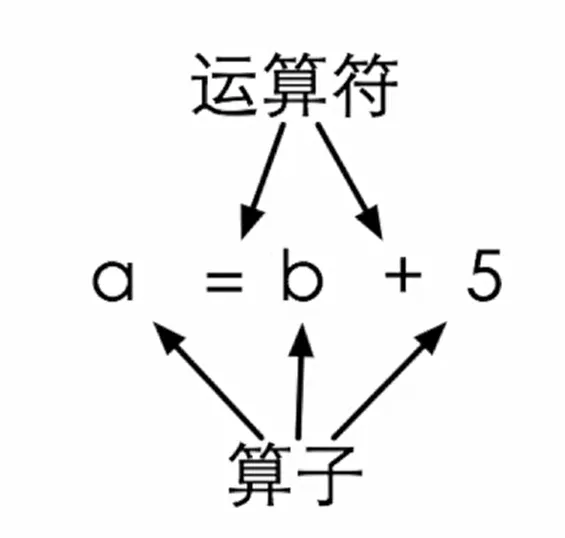# C 学习笔记 III

### 第一个 C 程序

``````#include<stdio.h>
int main()
{
printf("Hello World!");
return 0;
}``````

### 常量

``````int price = 0;

scanf("%d,&price");

int change = 100 - price;

printf("Take your change %d dollars \n",change);``````

``const int AMOUNT = 100;``

``````#include<stdio.h>
{
const int AMOUNT = 100;
int price = 0;

scanf("%d,&price");

int change = AMOUNT - price;

printf("Take your change %d dollars \n",change);

return 0;
}``````

Q：这样做有什么好处呢？

A：比如，别人第一次看这个 “100” 可能不知道是什么，但是看到“AMOUNT”可以知道这是跟数量（金额）有关

### const 修饰符

`const`是一个修饰符，像上面这样加在 `int` 前面，表示给这个变量加上一个`const`（不变的）属性：即这个变量的值一旦 `初始化`（赋初值），就不能再修改了！一般习惯性的在 `const` 后面的变量名使用大写。

``````#include<stdio.h>
{
const int AMOUNT = 100;
int price = 0;

AMOUNT = 90;

scanf("%d,&price");

int change = AMOUNT - price;

printf("Take your change %d dollars \n",change);

return 0;
}``````

``````#include<stdio.h>
{
int amount = 100;
int price = 0;

scanf("%d,&price");

scanf("%d",&amount);

int change = amount - price;

printf("Take your change %d dollars \n",change);

return 0;
}``````

### 浮点数

Q：美国人习惯使用英制计量单位，用几尺几寸来报自己的身高，如果遇到一个美国佬告诉你他是 5 英尺 7 寸，那他的身高是多少呢？

``````#include<stdio.h>
int main()
{
printf("请分别输入身高的英尺数和英寸数，如：1 2 \n");

int foot;
int inch;

scanf("%d,%d", &foot, &inch);
printf("他的身高是 %f 米\n",((foot+inch/12)*0.3048));

return 0;
}``````

• 两个整数运算结果只能是整数
• 比如：在 C 中，10/3=3
• 10 和 10.0 是两个不同的数

### 计算时间差

Q：输入两个时间，每个时间分别输入小时和分钟的值，然后输出两个时间的差：

``````int hour1, minute1;
int hour2, minute2;

scanf("%d %d"，&hour1, &minute1);
scanf("%d %d"，&hour2, &minute2);``````

• hour*60 + minute——>转化为 `minute` 单位
• t/60——> `hour` 部分
• t%60——> `minute` 部分

``````#include<stdio.h>

int main()
{
int hour1, minute1;
int hour2, minute2;

scanf("%d %d", &hour1, &minute1);
scanf("%d %d", &hour2, &minute2);

int t1 = hour1*60 + minute1;
int t2 = hour2*60 + minute2;

int t = t2-t1;

printf("时间差是%d小时%d分", t/60, t%60);

return 0;
}``````

### 运算符优先级1+单目不变自右向左a*+b
1-单目取负自右向左a*-b
2*自左向右a*b
2/自左向右a/b
2%取余自左向右a%b
3+自左向右a+b
3-自左向右a-b
4=赋值自右向左a=b

#### 赋值运算符

• a=8 ——> a 被赋予值（8）
• a=b=8 ——> a=(b=8)

#### “嵌入式赋值”

``````int a = 6;
int b;
int c = 1+(b=a);``````

#### 结合关系

• 一般自左向右
• 单目 +/- 和赋值 = 自左向右

``````result = a = b = 3 + c;
result = 2;
result = (result = result*2)*6*(result = 3 + result);``````

### 计算复利

Q：在银行存定期的时候，可以选择到期自动转存，并将到期的利息自动并入本金合并转存。如果一年的定期利率是 3.3%，那么连续转存三年后，最初存入的 x 元定期会得到多少本息余额？

``````int x;
printf("Plese input x:");
scanf("%d",&x);
double amount = x*(1+0,033)*(1+0.033)*(1+0.033)
printf("%f",amount);``````

### 交换变量

• 交换前：a = 2，b = 3，t

• 交换后：a = 3，b = 2，t

``````#include<stdio.h>

int main()
{
int a = 2;
int b = 3;
int t;

t = a;
a = b;
b = t;

printf("a=%d,b=%d",a,b);

return 0;
}``````

### 复合赋值

5个算术运算符：+、-、*、/、% 可以和 = 结合起来，形成`复合赋值运算符`：+=、-=、*=、/=、%=

• total += 5 ——> total = total + 5
• total += (sum+100)/2 ——> total = total + (sum+100)/2
• total *=sum + 12 ——> total = total * (sum+12)
• total /= 12 + 6 ——> total = total / (12+6)

### 递增/递减运算符

“++” 和 “--”是两个很特殊的运算符，它们是`单目运算符`，这个算子还必须是`变量`。这两个运算符分别叫做递增和递减运算符，它们的作⽤用就是给这个变量 +1 或者 -1

#### 前缀后缀

• ++ 和 -- 可以放在变量的前面，叫做 `前缀形式`，也可以放在变量的后面，叫做 `后缀形式`
• a++ 的值是 a 加 1 以前的值，⽽而 ++a 的值是加了1以后的值，无论哪个，a 自己的值都加了 1

count++给count的值加1count原来的值
++count给count的值加1count+1后的值
count--给count的值减1count原来的值
--count给count的值减1count-1后的值

``````#include<stdio.h>

int main()
{
int a;
a = 10;

printf("a++=%d\n",a++);
printf("a=%d\n",a);

printf("++a=%d\n",++a);
printf("a=%d",a);

return 0;
}``````

``````a++=10
a=11
++a=12
a=12``````

Dejavu

2020-08-02

2021-10-16